# Test: MCQs (One or More Correct Option): Mechanical Properties of Solids and Fluids | JEE Advanced

## 9 Questions MCQ Test Physics 35 Years JEE Main & Advanced Past year Papers | Test: MCQs (One or More Correct Option): Mechanical Properties of Solids and Fluids | JEE Advanced

Description
Attempt Test: MCQs (One or More Correct Option): Mechanical Properties of Solids and Fluids | JEE Advanced | 9 questions in 10 minutes | Mock test for JEE preparation | Free important questions MCQ to study Physics 35 Years JEE Main & Advanced Past year Papers for JEE Exam | Download free PDF with solutions
QUESTION: 1

### A body floats in a liquid contained in a beaker. The whole system as shown in Figure falls freely under gravity. The upthrust on the body is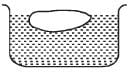Solution:

The whole system falls freely under gravity Upthrust = weight of fluid displaced
= (mass of fluid displaced) × g
For a freely falling body, g = 0
∴ Upthrust = 0.

*Multiple options can be correct
QUESTION: 2

### The spring balance A reads 2 kg with a block m suspended from it. A balance B reads 5 kg when a beaker with liquid is put on the pan of the balance. The two balances are now so arranged that the hanging mass is inside the liquid in the beaker as shown in the figure. In this situation: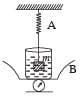Solution:

When the block of mass m is arranged as shown in the figure, an upthrust FT will act on the mass which will decrease the reading on A.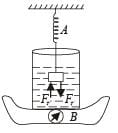Note :

According to Newton's third law, to each and every action, there is equal and opposite reaction.
So FT will act on the liquid of the beaker which will increase the reading in B.

*Multiple options can be correct
QUESTION: 3

### A vessel contains oil (density = 0.8 gm/cm3) over mercury (density = 13.6 gm cm3). A homogeneous sphere floats with half its volume immersed in mercury and the other half in oil. The density of the  material of the sphere in gm/cm3 is

Solution:

Weight of sphere = Upthrust due to Hg + Upthrust due to oil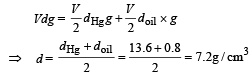*Multiple options can be correct
QUESTION: 4

Two rods of different materials having coefficients of thermal expansion α1, α2 and Young’s modulii Y1, Y2 respectively are fixed between two rigid massive walls. The rods are heated such that they undergo the same increase in temperature. There is no bending of the rods. If α1 : α2 = 2 : 3, the thermal stresses developed in the two rods are equal provided Y1 : Y2 is equal to

Solution: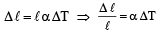Stress = Y × strain  ∴ Stress = Yα ΔT
For first  rod stress = Y1α1Δ T
For second rod stress = Y2α2ΔT
Since, stresses are equal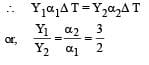*Multiple options can be correct
QUESTION: 5

Water from a tap emerges vertically downwards with an initial spped of 1.0 m s–1. The cross-sectional area of the tap is 10–4 m2. Assume that the pressure is constant throughout the stream of water, and that the flow is steady. The crosssectional area of the stream 0.15 m below the tap is

Solution:

KEY CONCEPT :
The equation of continuity is : v1A1 = v2A2 where v and A represent the speed of water stream and its area of cross section, respectively. We are given that

v1 = 1.0 ms–1
A1 = 10–4 m2
v2 = velocity of water stream at 0.15 m below the tap A2 = ?
For calculating v2
u = 1 m/s;  s = 1.5 m,  a = 10 m/s2  and  v = ?
v2 – u2 = 2as
v2 – 1 = 2 × 10 × 0.15
⇒ v = 2 m/s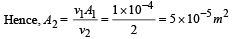*Multiple options can be correct
QUESTION: 6

A solid sphere of radius R and density ρ is attached to one end of a mass-less spring of force constant k. The other end of the spring is connected to another solid sphere of radius R and density 3ρ. The complete arrangement is placed in a liquid of density 2ρ and is allowed to reach equilibrium. The correct statement(s) is (are)

Solution:

Consider the equilibrium of the system of both spheres and the spring.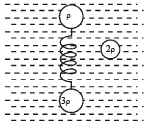The weight of system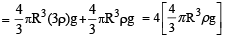This is to be balanced by the buoyant force.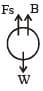This can be possible only when the light sphere is completely submerged. In this way the buoyant force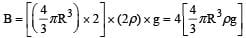Now considering the equilibrium of the heavy sphere Fs + B = W

∴ Fs = W – B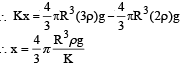*Multiple options can be correct
QUESTION: 7

In plotting stress versus strain curves for two materials P and Q,a student by mistake puts strain on the y-axis and stress on the x-axis as shown in the figure. Then the correct statement(s) is (are)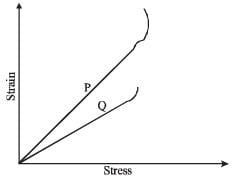Solution:

The maximum stress that P can withstand before breaking is greater than Q. Therefore (A) is a correct option.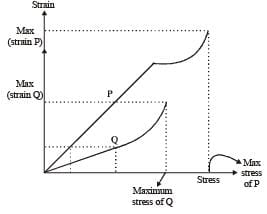The strain of P is more than Q therefore P is more ductile. Therefore (B) is a correct option.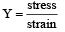For a given strain, stress is more for Q. Therefore YQ > YP.

*Multiple options can be correct
QUESTION: 8

A spherical body of radius R consists of a fluid of constant density and is in equilibrium under its own gravity. If P(r) is the pressure at r(r < R), then the correct option(s) is (are)

Solution:

Let us consider an elemental mass dm shown in the shaded portion.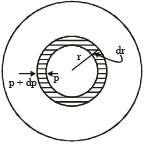Here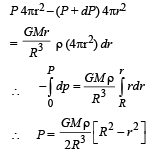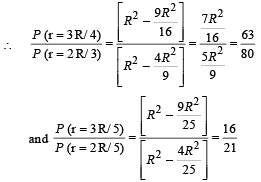B and C are correct options.

*Multiple options can be correct
QUESTION: 9

Two spheres P and Q of equal radii have densities ρ1 and ρ2, respectively. The spheres are connected by a massless string and placed in liquids L1 and L2 of densities σ1 and σ2 and  viscosities η1 and η2, respectively. They float in equilibrium with the sphere P in L1 and sphere Q in L2 and the string being taut (see figure). If sphere P alone in L2 has terminal velocity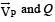alone in L1 has terminal velocity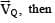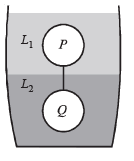Solution:

From the figure it is clear that
(a) σ2 > σ1
(b) ρ2 > σ2 [As the string is taut]
(c) ρ1 < σ1 [As the string is taut]
∴ ρ1 < σ1 < σ2 < ρ2

When P alone is in L2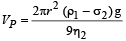is negative as ρ1 < σ2

Where r is radius of sphere.

When Q alone is in L1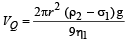is positive as ρ2 > σ1

Therefore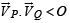option (d) is correct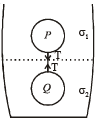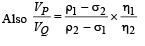...(i)

For equilibrium of Q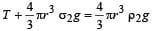...(ii)
For equilibrium of P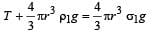...(iii)

(iii) – (ii) gives

ρ1 – σ2 = σ1 – ρ2 ...(iv)

From (i) and (iv)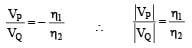∴ A is also a correct optionUse Code STAYHOME200 and get INR 200 additional OFF Use Coupon Code